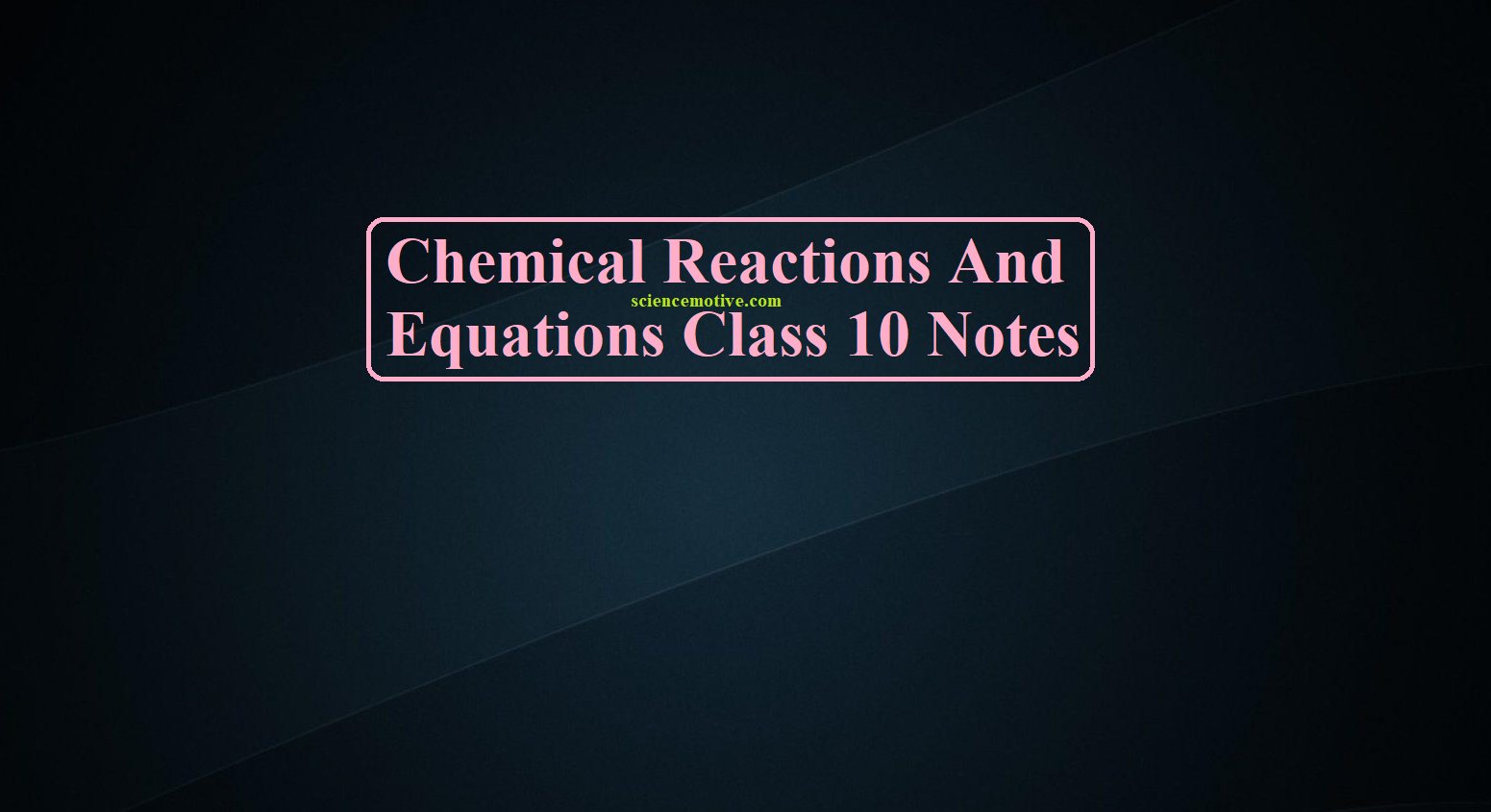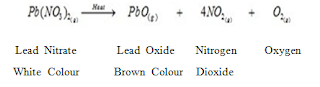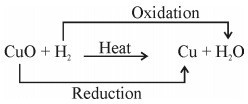# Chemical Reactions And Equations Class 10 Notes# Chemical Reactions And Equations Class 10 Notes

Chemical Reactions And Equations Class 10 Notes

Physical Change  Change in physical properties.

• Melting
• Boiling
• Condensation

Note- No change occurs in the identity of the substance

Chemical Change

• Atoms in the reactants are rearranged to form one or more different substances.
• Old bonds are broken, new bonds are formed.
• Reactants lose their properties to form products of different properties.

Examples –

• Cooking of food
• Rusting of iron
• Souring of milk
• Ripening of fruit.

Rusting of iron is a chemical change because

•  A new substance iron oxide is formed. The change is permanent; the article has got a rust layer (which may only peal off).
•  There is an increase in mass when rust forms.
• An energy change has taken place (which may not be visible).

4 Fe(s) +      3 O2 (g)    —>   2 Fe2O3 (rust)                 Iron            Oxygen                       Ferric oxide

Chemical reaction:

Chemical changes or chemical reactions are the changes in which one or more new substances are formed. e.g. Food gets digested in our body.

• Rusting of iron.
• Magnesium is burnt in air to form magnesium oxide. This chemical reaction can be represented as 2 Mg + O2  –>  2MgO
• We can observe or recognize a chemical reaction by observing changes in state, colour, the evolution of gas, or changes in temperature.
• the How do anabolic steroids work in the brain? | National Institute on Drug Abuse tri tren 250 a pharma merger’s impact ripples across west virginia as viatris plant closes | biopharma dive physical state of the reactants and the products are mentioned to make the chemical reactions more informative. e.g. we use (g) for gas, (l) for liquid, (s) for solid, and (aq) for aqueous.

Chemical Equations Representation of a chemical reaction in terms of symbols and formula of the reactants and products is known as a chemical equation.

Features of a chemical equation:

• The reactants are written on the left-hand side with a plus sign between them.
• The products are written on the right-hand side with a plus sign between them.
• An arrow separates the reactants from the products. The arrowhead points towards the products and indicates the direction of the reaction.

Skeletal chemical equation

• A chemical equation that simply represents the symbols and formulas of reactants and products taking part in the reaction is known as the skeletal chemical equation for a reaction.
• For example: For the burning of Magnesium in the air, Mg + O2 à MgO is the skeletal equation.

Balanced Equation

A balanced equation is one in which the number of atoms on the reactant and product sides is equal.

##### Or

The number of atoms of an element on the reactant side = number of atoms of that element on the product side.

• The simple form of representation of a chemical reaction in words is known as a word equation.
• Magnesium    +     Oxygen    ———–> Magnesium Oxide
• The representation of a chemical reaction with the help of chemical formula is called a chemical equation.
• 2Mg + O2→  2MgO

Writing Chemical Equations

• In a chemical reaction, the reactants are written on the left-hand side and the products on the right-hand side of the equation.
• An arrow (→) pointing towards the products is inserted between the reactants and the products. It also represents the direction of the reaction.
• A single arrow (→) indicates the direction in which the reaction proceeds.
• A double arrow( ↔ ) indicates a reversible reaction, i.e. the products recombine to form the reactants.
• A plus sign (+) is inserted between two or more reactants or products formed.
• If reactions are carried out under specific conditions of temperature, pressure, catalyst etc., then these conditions are mentioned on the arrow.
• The chemical equation can be made more informative by mentioning the physical states of the reactants and products.
• If gas is liberated as a product then it is represented by an arrow pointing upwards (↑). If the product formed is in the form of a precipitate, it is represented by an arrow pointing downwards (↓).

Steps Involved in Chemistry Balancing Chemical Equations Practice

Consider the chemical reaction between magnesium and oxygen to understand the steps involved in balancing a chemical equation. Step 1 Let us first write the word equation for this reaction. Magnesium + Oxygen→Magnesium oxide

Step 2 Write the chemical equation for the reaction between magnesium and oxygen. Mg + O2 → MgO

Step 3 Count the number atoms of an element occurring on both L.H.S. and R.H.S. in this equation. Mg + O2 → MgO

This is an unbalanced equation.

Step 4

#### To balance a chemical equation, first draw boxes around each formula. Do not change anything inside the boxes while balancing the equation.

• Choose a reactant or a product that has the maximum number of atoms in it. In that compound, select the element which has the maximum number of atoms. In this equation, we shall select MgO i.e. magnesium oxide, and the element oxygen in it.
• To balance the oxygen atoms, let us multiply the magnesium oxide molecule by 2 on the right-hand side. The equation can now be expressed as,

Step 5

There are two oxygen atoms on either side of the equation but one magnesium atom on the reactant’s side and two on the product’s side. Therefore, multiply the magnesium atom by 2 on the left-hand side.

A balanced equation is,

The number of atoms of each element of reactants = The number of atoms of each element of products

Step 6

Writing Specific Conditions on the Arrow

The reaction is carried out in the presence of ‘Heat’. On heating, magnesium combines with oxygen present in air to form magnesium oxide.

Step 7

Writing Symbols of Physical States

Using these steps, you can balance any chemical equation.

Chemistry Balancing Chemical Equations Practice :

Que1. Why do we need to balance a chemical equation?

Que2. Balance the following equations:

i)                Fe + H2O  —–> Fe2O3 + H2

ii)              Zn + HNO3   ——>  Zn(NO3)2 + H2O +  N2O

iii)             NaHCO3 ——> Na2CO3 + H2O + CO2

Chemical Reactions And Equations Class 10 Notes

Types of Chemical Reactions

i). Combination Reaction

ii). Decomposition Reaction

a). Thermal Decomposition

b). Electrolytic Decomposition

iii). Displacement Reaction OR Substitution reaction

iv). Double Displacement Reaction

a). Precipitation Reaction

b). Neutralisation Reaction

v).  Redox Reaction

vi). Oxidation Reaction

vii). Reduction Reaction

viii). Endothermic Reaction

ix). Exothermic Reaction

Explanation of the types of Chemical reactions i.e Combination, Decomposition, Displacement And Double Displacement reactions using examples:

i). Combination Reaction:

The reactions in which two or more substances combine to form a new substance are called combination reactions. For example: a).  2 Mg (s)    +     O2 (g)     →  2 MgO

Magnesium      Oxygen         Magnesium oxide (White ash)

(basic) turns red litmus blue

b). In the laboratory, iron sulphide is prepared by mixing iron and sulphur.

Fe(s)   + S(s)    →   FeS(s)

c). Burning of carbon monoxide in oxygen to form carbon dioxide.

2CO (g)       +   O2(g)  →       2CO2 (g)

d).  Calcium oxide upon reaction with water produces calcium hydroxide.

CaO                +            H2O        →    Ca(OH)2

Calcium Oxide       Water           Calcium Hydroxide

(Quick Lime)                                (Slaked Lime)

Chemical Reactions And Equations Class 10 Notes

ii). Decomposition reactions:

These are opposite to combination reactions. A single compound decomposes or breaks down to give two or more simpler substances. It is of the following types:

I). Thermal Decomposition: When a decomposition reaction is carried out by heating For Example:

• Mercuric oxide, when heated, undergoes thermal decomposition, to give mercury and oxygeniii). Displacement Reaction OR Substitution reaction

A more reactive element (metal) displaces less reactive element (metal) from its aqueous salt soln. For example:

• Zn (s)  +   FeSO4 (aq)    →    ZnSO4 (aq) + Fe (s)

(green)               (Colourless)

• Fe (s)  +  CuSO4 (aq)    →    FeSO4 (aq)  + Cu (s) ↓

Iron        Blue                   Green            Reddish Brown

• Mg (s)        +    H2SO4 (aq)     →   MgSO4 (aq) +  H2O (g)

Magnesium    Sulphuric             Magnesium     Water

Acid                        Sulphate

• Cu (s)     +     2AgNO3 (aq)      →   Cu (NO3)2 (aq)    +   2Ag (s).

Copper            Silver Nitrate      Copper Nitrate      Silver

• KI (aq)      +    Cl2 (g)           →  KCl (aq)    +    I2 (g)

Potassium     Chlorine           Potassium    Iodine

Iodide                                          Chloride

iv). Double Displacement Reaction:

The chemical reactions in which compounds react to form two different compounds by mutual exchange of ions are called double displacement reactions.

Reactions occur by two different ways

a). Precipitation: In such reactions due to the exchange of ions some insoluble material is formed. This insoluble material is called precipitate and the reaction is called a precipitation reaction. For example:

• ZnSO4 (aq)    +   BaCl2 (aq)     →   ZnCl2 (aq)   +  BaSO4 (s)

Zinc                  Barium                 Zinc                Barium

Sulphate             Chloride              Chloride        Sulphate (White)

• 2 HCl (aq)      +   Pb(NO3)2(aq) →  2 HNO3 (aq)  +  PbCl2 (s)

Acid                        Nitrate                    Acid                Chloride

• Pb(NO3)2(aq)  +   2 KI (aq)        →    PbI2 (aq)   +   KNO3 (s)

Nitrate                    Iodide                 Iodide              Nitrate

b) Neutralization Reaction: In this type of reaction an acid reacts with a base to form salt and water by exchange of ions.

• NaOH (aq)   +  HCl (aq)       →      NaCl (aq)     +    H2O

Sodium          Hydrochloric       Sodium             Water

Hydroxide      Acid                        Chloride

(Base)             (Acid)                      (Salt)                (Water)

• ZnO     +     HNO3    →   Zn(NO3)2   +   H2O

Zinc            Nitric           Zinc                 Water

Oxide         Acid             Nitrate

Chemical Reactions And Equations Class 10 Notes

Redox Reaction, Oxidation and Reduction Reactions

This part of the types of chemical reaction of chapter Chemical Reactions and Equations involve the types Redox Reaction, Oxidation and Reduction Reactions with exact equations.

v). Redox Reaction: A reaction in which reduction and oxidation takes place simultaneously.• ZnO (s)    +    C (s)      →   Zn (s)    +   CO (g)

Zinc              Carbon      Zinc           Carbon

Oxide                                                  Monoxide

vi). Oxidation Reaction: Oxidation is the gain of oxygenFor example:

• 2 Cu (s)   +    O2 (g)     →    2 CuO (s)  (Black)

Copper        Oxygen           Copper Oxide

•  2 Mg(s)    +   O2 (g)      →    2MgO (s)

Magnesium   Oxygen       Magnesium Oxide

OR

Removal of hydrogen is also oxidation. For Example:

•   2HI (g)          →    H2  (g)     +    I2 (g)

Hydrogen          Hydrogen    Iodine

Iodic Acid

VII). Reduction Reaction: Reduction is the gain of hydrogenFor example:

•   H2 (g)     +      Cl(g)  →    2 HCl

Hydrogen    Chlorine      Hydrochloric Acid

OR

Removal of oxygen is also reduction. Foe Example:

•   CuO (s)       +      CO (g)       →      Cu (s)     +     CO2 (g)

Copper Oxide   Carbon                Copper          Carbon Dioxide

(Black)             Monoxide           (Reddish Brown)

•   ZnO (s)    +    C (s)      →   Zn (s)    +   CO (g)

Zinc              Carbon        Zinc            Carbon

Oxide                                                     Monoxide

An oxidation-reduction (redox) reaction is a type of chemical reaction that involves the transfer of electrons between two species. An oxidation-reduction reaction is any chemical reaction in which the oxidation number of a molecule, atom, or ion changes by gaining or losing an electron. Redox Reaction, Oxidation, and Reduction Reactions with exact equations are common and vital to some of the basic functions of life, including photosynthesis, respiration, combustion, and corrosion or rusting.

1.   Oxidation: The process in which there is loss of electrons.
2.   Reduction: The process in which there is a gain of electrons.
3.   Oxidizing Agent: A substance that brings about oxidation.
4.  Reducing Agents: A substance that brings about reduction.

viii). Endothermic Reactions: Reaction in which heat is absorbed to carry out the chemical reaction. For Example:

•        CaCO3  (s)      +      Heat      →      CaO (s)      +      CO2  (g)                                                                                                           Calcium                                           Calcium           Carbon

Carbonate                                      Oxide               Dioxide

•   C(s)    +  H2 O (s)  +    Heat  →    CO (g)    +    H2 (g)

Carbon                                             Carbon

Monoxide (Evolve)

ix). Exothermic Reactions: Reaction in which heat is evolved during the chemical reaction. For Example:

•   C    +    O2    →     CO2 (g)    +     heat

Chemical Reactions And Equations Class 10 Notes

The Effect of Oxidation-Reduction in Daily Life Corrosion

Many metals are chemically active elements and get easily affected by substances like moisture, air, acids, etc, and undergo Oxidation, Reduction in Daily Life.

1. Corrosion – The process of slow conversion of metals into their undesirable compounds due to their reaction with oxygen, water, acids, gases, etc. present in the atmosphere is called corrosion.
2. Rusting of Iron: We know iron articles that are shiny when new and get coated with a reddish-brown powder when left for some time this process is called Rusting of Iron. Chemically, Rust is a hydrated ferric oxide (Fe2O3.xH2O)
• Advantages of Corrosion: Though corrosion is undesirable, it can be advantageous in the case of aluminum which on exposure to air, gets coated with a protective layer of aluminum oxide. This protects the metal underneath from further corrosion and damage.
• The black coating on silver and the green coating on copper are examples of corrosion in which the oxides formed a strong bond to the surface of the metal, preventing the surface from further exposure to oxygen and consequently slowing down corrosion.
• Rusting of iron can be prevented by paintingoiling the surface, or by galvanization.
• Rancidity:  When oils and fats or foods containing oils and fats are exposed to air, they get oxidized due to which the food becomes stale (no longer fresh and pleasant to eat; hard or dry) and gives a bad taste or smell this is called Rancidity. The most important cause of rancidity is the deterioration in fats and fatty foods because of the oxidation process. When an oxygen atom replaces a hydrogen atom in the fatty acid molecule it destabilizes the molecule. Factors that accelerate fat oxidation include salt, light, water, bacteria, molds trace metals (iron, zinc, etc.). Above mentioned topics are important examples of Oxidation-Reduction in Daily Life

It can be prevented by using various methods such as by adding antioxidants to the food materials, Storing food in an airtight container, and flushing out air with nitrogen.

What you have learned

•  A complete chemical equation represents the reactants, products, and their physical states symbolically.
•  A chemical equation is balanced so that the numbers of atoms of each type involved in a chemical reaction are the same on the reactant and product sides of the equation. Equations must always be balanced.
• In a combination reaction, two or more substances combine to form a new single substance.
• Decomposition reactions are opposite to combination reactions. In a decomposition reaction, a single substance decomposes to give two or more substances.
• Reactions in which heat is given out along with the products are called exothermic reactions.
• Reactions in which energy is absorbed are known as endothermic reactions. When an element displaces another element from its compound, a displacement reaction occurs.
• Two different atoms or groups of atoms (ions) are exchanged in double displacement reactions.
• Precipitation reactions produce insoluble salts.

Oxidation-Reduction in Daily Life also involves the gain or loss of oxygen or hydrogen by substances. Oxidation is the gain of oxygen or loss of hydrogen. The reduction is the loss of oxygen or the gain of hydrogen.

Chemical Reactions And Equations Class 10 Notes